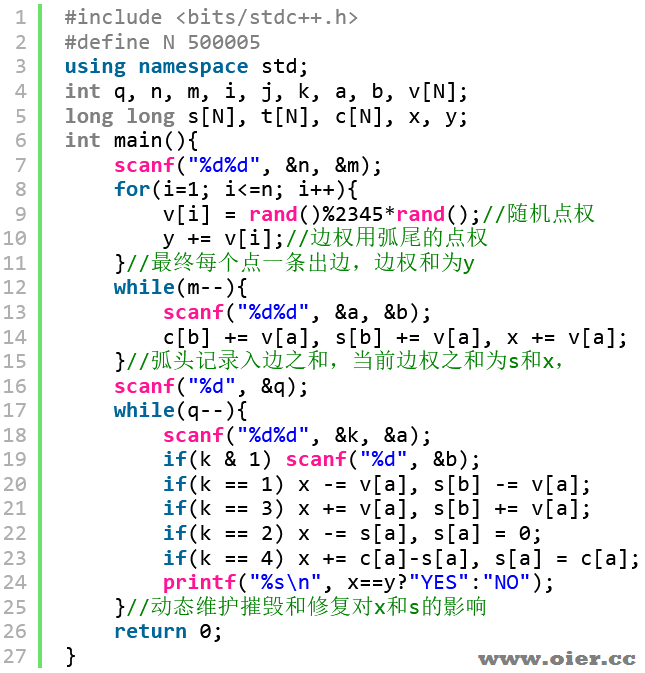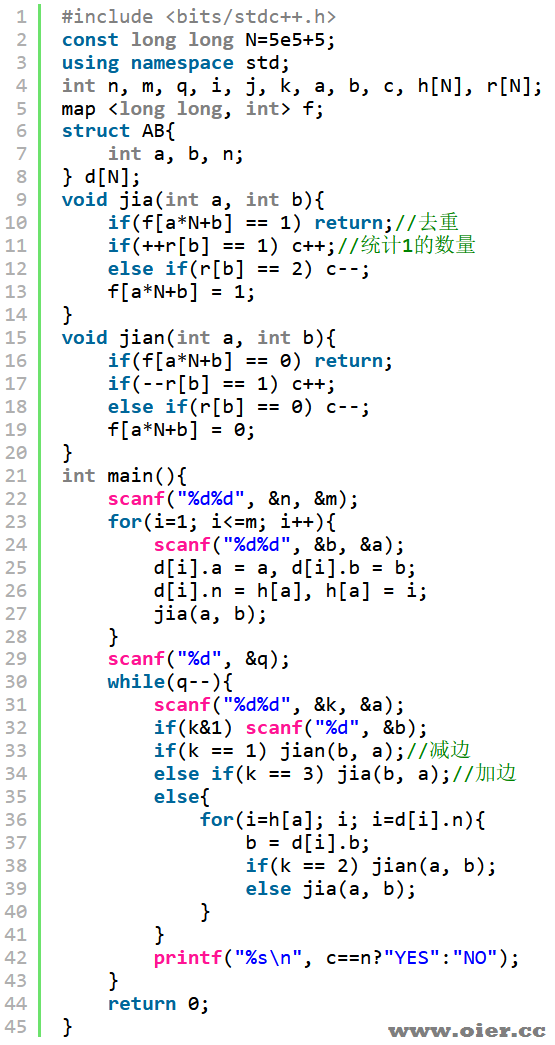602+

## 题目描述

1. 敌人会摧毁某个虫洞，这会使它连接的两个据点无法再通过这个虫洞直接到达，但这样的打击无法摧毁它连接的两个据点。
2. 敌人会摧毁某个据点，由于虫洞的主要技术集中在出口处，这会导致该据点的所有还未被摧毁的虫洞被一同摧毁。而从这个据点出发的虫洞则**不会摧毁**。

– A 型特种部队则可以将某个特定的虫洞修复。
– B 型特种部队可以将某据点的所有损坏的虫洞修复。

– 如果从我方的任何据点出发，在选择了合适的路线的前提下，可以进行无限次的虫洞穿梭（可以多次经过同一据点或同一虫洞），那么这个据点就可以**实现反击**。
– 为了使虫洞穿梭的过程连续，尽量减少战舰在据点切换虫洞时的质能损耗，当且仅当**只有一个从该据点出发的虫洞可用**时，这个据点可以**实现连续穿梭**。
– 如果我方所有据点都可以**实现反击**，也都可以**实现连续穿梭**，那么这个时刻就是一个绝佳的**反攻**时刻。

## 输入输出格式

### 输入格式

– 若 $t = 1$，接下来两个整数 $u, v$ 表示敌人摧毁了从据点 $u$ 出发到据点 $v$ 的虫洞。保证该虫洞存在且未被摧毁。
– 若 $t = 2$，接下来一个整数 $u$ 表示敌人摧毁了据点 $u$。如果该据点的虫洞已全部 被摧毁，那么这次袭击不会有任何效果。
– 若 $t = 3$，接下来两个整数 $u, v$ 表示我方修复了从据点 $u$ 出发到据点 $v$ 的虫洞。保证该虫洞存在且被摧毁。
– 若 $t = 4$，接下来一个整数 $u$ 表示我方修复了据点 $u$。如果该据点没有被摧毁的虫洞，那么这次修复不会有任何效果。

## 输入输出样例

### 输入样例 #1

3 6
2 3
2 1
1 2
1 3
3 1
3 2
11
1 3 2
1 2 3
1 1 3
1 1 2
3 1 3
3 3 2
2 3
1 3 1
3 1 3
4 2
1 3 2


### 输出样例 #1

NO
NO
YES
NO
YES
NO
NO
NO
YES
NO
NO


## 说明

**【样例解释 \#1】**

**【样例 \#2】**

**【样例 \#3】**

**【样例 \#4】**

**【数据范围】**

| 测试点 | $n \le$ | $m \le$ | $q \le$ | 特殊限制 |
| :———–: | :———–: | :———–: | :———–: | :———–: |
| $1 \sim 3$ | $10$ | $20$ | $50$ | 无 |
| $4 \sim 8$ | ${10}^3$ | ${10}^4$ | ${10}^3$ | 无 |
| $9 \sim 10$ | $5 \times {10}^5$ | $5 \times {10}^5$ | $5 \times {10}^5$ | 保证没有 $t = 2$ 和 $t = 4$ 的情况 |
| $11 \sim 12$ | $5 \times {10}^5$ | $5 \times {10}^5$ | $5 \times {10}^5$ | 保证没有 $t = 4$ 的情况 |
| $13 \sim 16$ | ${10}^5$ | $5 \times {10}^5$ | $5 \times {10}^5$ | 无 |
| $17 \sim 20$ | $5 \times {10}^5$ | $5\times 10^5$ | $5 \times {10}^5$ | 无 |

## 程序实现